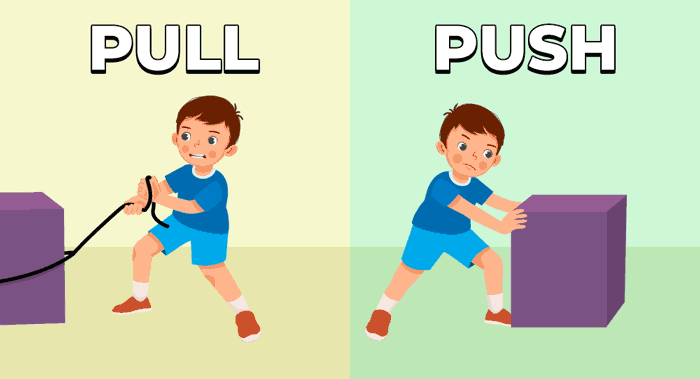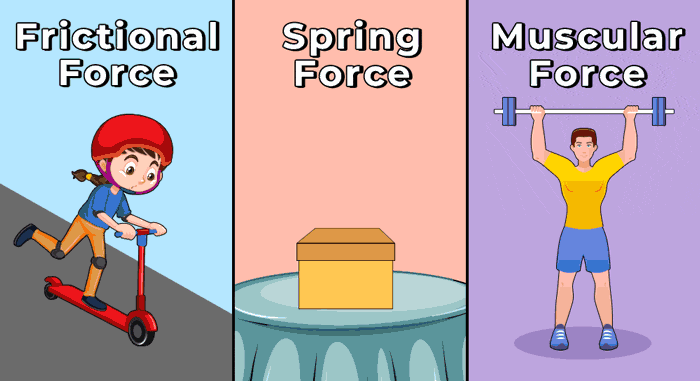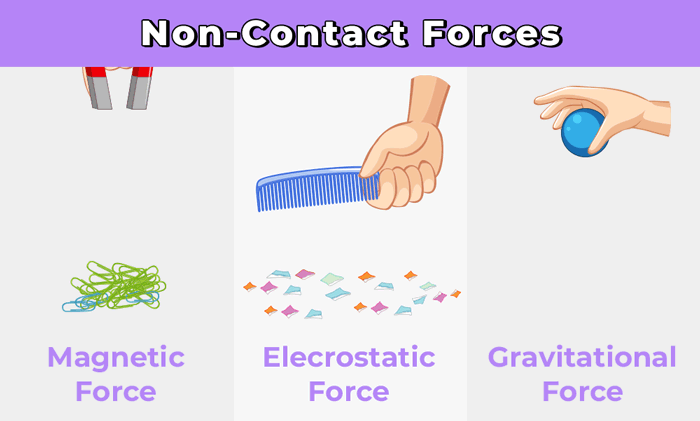GFG App
Open AppBrowser
Continue

Force is defined as an external cause that a body experiences as a result of interacting with another body. Whenever two objects interact, a force is exerted on each object.

In general-term “To Push or Pull an Object” is defined as the force. The force is the interaction experience by the object because of the other object. Generally, force applied to an object changes its state of rest or motion and it also adds acceleration to the object.

## What is Force?

A push and pull of an object are referred to as a force in science. Force is created when two things interact. The force is a vector quantity as it has both magnitude and direction. The magnitude of a force is represent its strength. The force can also cause a change in direction of the body to which the force is applied.

Any external element that has a tendency to change the state of motion or rest, when applied to an object is called force. Force is simply the push or pull upon an object resulting to the interaction between the two objects.

A force can cause an item to move from rest to motion or vice versa. Two or more things must interact with each other for a force to be created. For example, a force is applied by the wind when it propels a sailboat through the water, A force is also present when gravity pulls an apple downward. Things can move, change their speed, or change shape in response to forces.## Unit of Force

• Force is a vector quantity, this implies it has both mass and magnitude. The force acting on an object is represented by the symbol F or.
• The SI unit of force is Newton (N). It is named after the famous English scientist Sir Issac Newton. 1-Newton force is defined as the force required to accelerate a mass of 1 kg by 1 m/s2 in the direction of applied force.
• In the CGS system, the unit of force is Dyne.

### Dimension Of Force

Force is defined as the product of the mass and acceleration of the body. So its dimensional formula is, [MLT-2].

## Effects of Forces

When the force is applied to an object then it has different effects on the object like,

• Force can Change the Shape and Size of an Object
• Force can Change the direction of an Object
• Force can Change the Speed of an Object
• Force can change the state of Rest or Motion of an Object

Let’s understand the effects of force and its example

### Force can Change the Shape and Size of an Object

Force will cause a change in shape, for example when hammering a hot iron rod, the force exerted by the hammer will change the object’s shape, i.e. iron rod will be bent.

Force will cause a change in size, by stretching a rubber band, the force exerted by stretching a rubber band will cause an increase in the size of the rubber band.

### Force can Change the direction of an Object

Force will cause a change in direction, for example, hitting a football, the time you kick a ball, then its direction will get changed.

### Force can Change the Speed of an Object

When a batsman hit the moving ball with force will cause an increase in the speed of the ball. Also, the speed of force will depend on which direction it is applied, if we apply a force in the direction of a moving object then it will cause an increase in speed and when it applies against the moving object then it will decrease the speed of an object.

### Force can change the state of Rest or Motion of an Object

Force will cause a change in motion, for example, the force exerted by the motor of the car will enable the car to move, while force exerted by the brake will enable the car to stop.

## Force Formulas

Force is defined by the product of the mass and acceleration of the object. For a body with mass “m” and the acceleration “a” the force can be calculated as,

F = ma

where,

F is the applied force,
m is the mass of an object and
a is the acceleration of the object.

Also according to Newton’s Second Law of Motion, force is given by the time rate of change of momentum. Mathematically, it is given as,where,

•is the change in momentum
• dt is the change in time.

But, momentumis defined as the product of mass and velocity of the object as,where

• m is the mass and
•is the velocity of the object.

Therefore, the force becomes:## Magnitude and Direction of Force

To move a large weight, the individual must pull or push it in the same direction. When two individuals push or pull weight in opposite directions, the resultant force is the sum of the two forces. The magnitude of a force expresses its strength. When forces are applied in the opposite direction, the size of the resultant force is reduced.

Furthermore, equal and opposing forces cancel out, thus the resulting force is zero. Force exerted on an item causes a change in velocity as well as a change in shape. Some forces operate on an item by making touch with it, whilst others act without making contact with the object. Touch forces are forces that act when an item comes into contact with them.

Contact forces include muscular forces and friction forces. Non-contact forces are those that may act without coming into touch with an item. Gravitational force, electromagnetic force, electrostatic force, and non-contact forces are all examples of forces

## Types of Force

A Force can act on an object with or without any contact. For example, if you are pushing or pulling a door then you must have contact with a door while if you have a bar magnet you can easily attract iron nails without having any contact with the nail. So in science, we have two types of forces, one is a contact force and the other is a non-contact force.

Let’s see in detail what are they with their examples.

## Contact Force

The forces which are applied to other objects only through physical touching are called contact forces.Examples of contact forces are muscular and frictional forces.

### Muscular Force

Muscular force is a contact force in which force is exerted by the muscles of the body. For example, jumping, kicking, running, walking, climbing, lifting, and pushing all are exerted by our muscles.

### Force of Friction

Frictional Force is a contact force that always opposes the body’s state of motion over another body. For example, if we stop paddling a bicycle, gradually it gets slows down and stops after covering some distance.

## Non-Contact Force

The forces which are applied to other objects without any physical touching are called non-contact forces.

Examples of non-contact forces are magnetic force, electrostatic force, and gravitational force. Now let’s discuss types of non-contact force in detail.### Magnetic Force

Magnetic force is a non-contact force exerted by a magnet on any other magnetic substance. For example, if we bring a magnet near an iron nail then the magnet pulls them towards it since magnets exert force.

### Electrostatic Force

An electrostatic force is a non-contact force that can be exerted by a charged object on another object from a distance. For example, when a plastic comb is rubbed in dry hair, then the electrically charged comb attracts a tiny piece of papers

### Gravitational Force

The Gravitational force is a non-contact force between two bodies having some mass. It is an attractive force. The force of attraction between the earth and any object is called the force of gravity.

## Line of Action of a Force

Galileo used experiments to demonstrate that when there is no external force acting on an object, it moves at a constant speed. He could observe that a sphere’s speed rises as it rolls down an inclined plane because of its gravitational attraction on it.

The net force acting on an object is 0 when all the forces are equal and balanced. A net force acting on a body, however, can change either the magnitude or the direction of its velocity if all of the forces acting on it result in an unbalanced force, which indicates that the unbalanced force can accelerate the body. For instance, when a body is subjected to a number of forces and is determined to be at rest, we can infer that there is zero net force operating on the body.

The line of action of a force is the path it takes as it exerts its force on an object. The point of application of the force is the location where it is exerting its force on an object. The force of friction is the force that opposes the relative motion between the surfaces of two objects in contact and acts along the surfaces.

## Summary

The basic summary of force can be understood with the help of the table discussed below.

Related Resources

## Solved Examples on Force

Example 1: Determine the force of an object with a mass equal to 500 kg and acceleration as 60 m/s2.

Solution:

Given,

Mass of the object, m = 500 kg.

Acceleration, a = 60 m/s2

According to force formula,

F = ma

Substituting the given values,

F = 500 kg × 60 m/s2

= 3 × 104 N

The required force is 3 × 104 N

Example 2: How much net force is required to accelerate to a 20 kg box at 5 m/s2?

Solution:

Given,

Mass of the box, m = 20 kg.

Acceleration of the box, a = 5 m/s2

According to force formula,

F = ma

Substituting the given values,

F = 20 kg × 5 m/s2

= 100 N

The required force is 100 N

Example 3: Find the acceleration of the object if the force applied is 250 N and the mass of the object is 50 kg.

Solution:

Given

Force applied is 250 N

Mass of the object, m = 50 kg

Acceleration of the object, a =?

According to force formula,

F = ma

Substituting the given values,

250 = 50 kg × a

a = 250/50

= 5 m/s2

Thus, the acceleration of the object is 5 m/s2

Example 4: Find the mass of the object if the force applied is 220 N and the acceleration of the object is 15 m/s2.

Solution:

Given

Force applied is 225 N

Mass of the object, m =?

Acceleration of the object, a = 15 m/s2

According to force formula,

F = ma

Substituting the given values,

225 = m × 15

m = 225/15

= 15 kg

Thus, the mass of the object is 15 kg

## FAQs on Force

### Q1: What is Force?

To Push Or Pull an object is defined as the force. Force is the interaction between two objects that tend to change the state of the object.

### Q2: What is the SI unit of force?

The SI unit of Force is Newton. One Newton is defined as the force applied when one kg object accelerates with 1 m/s2

### Q3: What force is friction?

Friction is a contact force which acts only when two objects are in contact with each other. It is an opposing force i.e. it always opposes the motion of the object.

### Q4: What can force do?

Force can do various things such as,

• Force can change the direction of the object.
• Force can change the state of rest or motion.
• force can change the speed of the object.
• Force can change the shape and size of the object.

### Q5: How many types of forces are there?

On the basis of interaction between two objects force can be categorised into two categories,

• Contact Force
• Non-Contact Force

### Q6: Which is the weakest force in nature?

Among all the fundamental forces “Gravitational Force” is the weakest force and is also considered to be the weakest force in nature.